﻿ 可燃冰沉积物力学特性的离散元模拟分析

# 可燃冰沉积物力学特性的离散元模拟分析Discrete Element Simulation Analysis of Mechanical Behavior of the Gas Hydrate-Bearing Sediments

Abstract: Pore-filling type of combustible ice-bearing sediment can be regarded as a class of mixed granular material forming with sand and hydrate particles, which is a typically discontinuous problem. In view of discontinuous problem, this paper proposed a new technique for generating pore-filling type of combustible ice-bearing sediment by the particle flow software PFC3D. A series of numerical simulations of triaxial compression simulation experiments are performed on gas hydrate bearing sediments to investigate the mechanical properties of combustible ice-bearing sediment under different confining pressure. The study shows that the DEM simulation for preparing sample is able to capture the mechanical characteristics of combustible ice-bearing sediment. The initial elastic modulus, peak stress peak strain and residual strength of combustible ice-bearing sediment increase upon the increased confining pressure. The trend of the change of stress-strain curves is similar. The peak strain of combustible ice-bearing sediment increases with the confining pressure increasing when the value of combustible ice saturation is same. The peak strain of combustible ice-bearing sediment increases linearly with the confining pressure increasing when the value of combustible ice saturation is different. The increase of confining pressure helps to improve the compressive deformation capacity of combustible ice-bearing sediment.

1. 引言

2. 离散元法的基本原理

DEM是Cundall  在1971年提出的，其基本思想是把物质描述为由一系列离散刚性单元构成的集合体，单元可以平移或转动，单元间可以接触或分离。基于牛顿第二运动定律建立单元的运动方程，利用显式中心差分法求解运动方程。物质的变形或破裂过程，由刚性单元运动及其相互位置来描述。由于刚性单元在运动中不存在变形协调问题，因此DEM特别适用于模拟固体材料大变形或破裂问题。图2为单元接触示意图，在DEM模拟计算中单元间处于接触还是分离，是通过单元间的重叠相对位移δ来判断的。图3为单元接触力学模型，单元间的相互作用一般用弹簧、粘壶及滑块进行定量描述。通常由法向刚度系数为kn和切向刚度系数为ks的两个弹簧，法向阻尼系数为βn和切向阻尼系数为βs的两个粘壶，摩擦系数为的一个滑块及代表单元与其他单元之间非接触的法向连接Tn和切向连接Ts组成。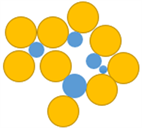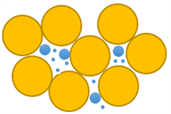(a) 孔隙填充型 (b) 骨架支撑型 (c) 颗粒胶结型

Figure 1. Form of combustible ice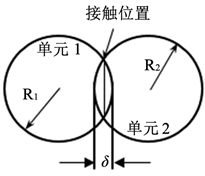Figure 2. Chart: contact between elements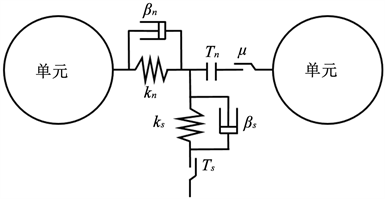Figure 3. Chart: mechanical model of contact between elements

${F}_{n}={K}_{n}{\delta }_{n}$ (1)

$\text{Δ}{F}_{s}={K}_{s}\Delta {\delta }_{s}$ (2)

$\left\{\begin{array}{l}{F}_{i}-{\beta }_{g}{\stackrel{˙}{u}}_{i}=m{\stackrel{¨}{u}}_{i}\left({t}_{0}\right)\\ {M}_{i}-{\beta }_{g}{\stackrel{˙}{\omega }}_{i}=I{\stackrel{¨}{\omega }}_{i}\left({t}_{0}\right)\end{array}$ (3)

$\left\{\begin{array}{l}{\stackrel{˙}{u}}_{i}\left({t}_{1}\right)={\stackrel{˙}{u}}_{i}\left({t}_{0}-\frac{\Delta t}{2}\right)+{\stackrel{¨}{u}}_{i}\left({t}_{0}\right)\Delta t\\ {\stackrel{˙}{\omega }}_{i}\left({t}_{1}\right)={\stackrel{˙}{\omega }}_{i}\left({t}_{0}-\frac{\Delta t}{2}\right)+{\stackrel{˙}{\omega }}_{i}\left({t}_{0}\right)\Delta t\end{array}$ (4)

$\left\{\begin{array}{l}{u}_{i}\left({t}_{2}\right)={u}_{i}\left({t}_{0}\right)+{\stackrel{˙}{u}}_{i}\left({t}_{1}\right)\Delta t\\ {\omega }_{i}\left({t}_{2}\right)={\omega }_{i}\left({t}_{0}\right)+{\stackrel{˙}{\omega }}_{i}\left({t}_{1}\right)\Delta t\end{array}$ (5)

3. 可燃冰沉积物DEM试样制备

3.1. 试样制备方法

1) 同时生成砂粒和可燃冰颗粒。与文献   中先生成砂粒再生成可燃冰颗粒的制备方法不同，本文同时生成满足初始孔隙率及饱和度要求的砂粒和可燃冰颗粒。具体实现过程为：① 将可燃冰颗粒的粒径设置为一较小的定值，设定砂粒粒径的取值范围；② 根据饱和度、初始孔隙率及砂粒粒径级配曲线，计算可燃冰沉积物孔隙率及其粒径级配曲线；③ 根据可燃冰沉积物孔隙率及粒径级配曲线，同时生成试样所需的砂粒和可燃冰颗粒。这种做法一方面能够确保可燃冰颗粒随机填充到砂粒中，真实反映可燃冰在沉积物中的分布情况；另一方面省略了繁琐的可燃冰颗粒数目计算过程，且可确保可燃冰沉积物的孔隙率及饱和度满足要求。

2) 调整颗粒间的重叠量。为了保证模拟的准确性，将线性接触模型赋予初始的DEM试样，使所有颗粒充分运动，监控颗粒间接触力使其重叠量达到合理范围内。为保证DEM试样的完整性，避免颗粒飞溅溢出，颗粒运动一定时步后对颗粒的运动状态清除重置。

3) 施加固结压力。为模拟可燃冰沉积物初始应力状态，对DEM试样施加一定的固结压力(本文取0.5 MPa)，使颗粒在固结压力下相互接触，最终达到平衡。

4) 赋予新的接触模型。在砂粒和可燃冰颗粒间以及可燃冰颗粒间的接触点赋予平行胶结模型。由于模拟采用的圆球颗粒与真实可燃冰的存在形式不同，DEM模拟中的材料微观参数往往难以确定。本文根据已有研究中的参数进行试算取值，最终得到的接触参数以及其他材料参数如表1所示。

$\alpha =\frac{V-{V}_{s}}{V}$ (6)

$\alpha \text{'}=\frac{V-{V}_{s}-{V}_{mh}}{V}$ (7)

${S}_{mh}=\frac{{V}_{mh}}{V-{V}_{s}}×100%$ (8)Table 1. Material properties used in DEM simulations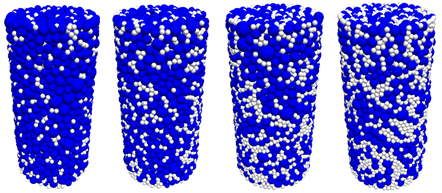Figure 4. DEM samples of combustible ice with saturation of 10%, 20%, 30% and 40.9%

3.2. 粒径级配曲线

${T}_{si}=\frac{{V}_{si}}{{V}_{s}+{V}_{mh}}\text{\hspace{0.17em}}\text{\hspace{0.17em}}\left(i=1,2,\cdots ,n\right)$ (9)

${T}_{mh}=\frac{{V}_{mh}}{{V}_{s}+{V}_{mh}}$ (10)

4. DEM数值模拟分析

4.1. 数值模拟过程

DEM模拟可燃冰沉积物三轴压缩试验过程为：① 利用PFC3D的伺服控制技术控制试验围压保持某一恒定状态；② 利用上、下墙体的移动对DEM试样进行轴向加载。为避免瞬时加载速度过大导致颗粒飞溅，造成模拟结果不准确，对上、下墙体速度采取逐级增加的加载方式，其速度和时步的关系曲线如图6所示。按照上述试验过程和速度加载方式，分别对不同饱和度和不同围压下的可燃冰沉积物试样进行了三轴压缩DEM数值模拟试验。模拟了不同饱和度和围压下可燃冰沉积物的力学行为，分析了饱和度和围压对可燃冰沉积物力学特性的影响。

4.2. 数值模拟分析(a) SMH = 10%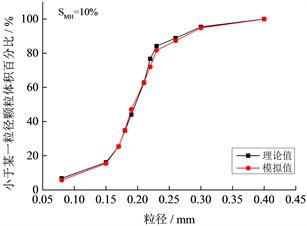(b) SMH = 20%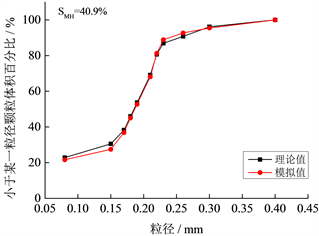(c) SMH = 30%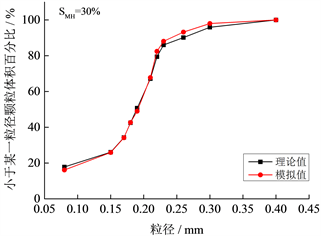(d) SMH = 40.9%

Figure 5. Curve: particle size distributions of combustible ice with different saturation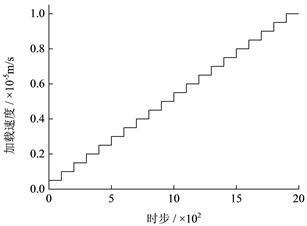Figure 6. Diagram of triaxial compression numerical simulation tests and loading curve(a) SMH = 10%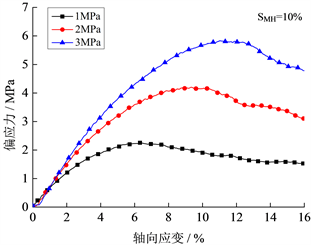(b) SMH = 20%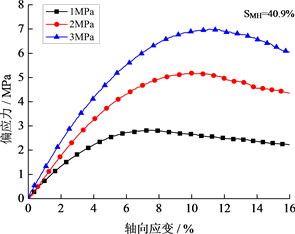(c) SMH = 30%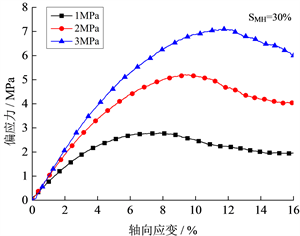(d) SMH = 40.9%

Figure 7. Curve: stress-strain relationship between combustible ice sediments under different confining pressuresFigure 8. Curve: peak strain-confining pressures relationship between combustible ice sediments under different saturation

5. 结论

1) 本文提出了一种制备孔隙填充型可燃冰沉积物DEM试样的新方法。该方法简单易行、重复性好，可确保可燃冰颗粒随机填充到砂粒中，能有效反映可燃冰的分布情况，且省略了已有方法的繁琐的可燃冰颗粒数目计算过程。通过与室内试验对比可知，本文的DEM模拟方法能够较好地反映可燃冰沉积物的力学特性。

2) 围压是影响可燃冰沉积物试样力学特性的重要因素。在相同饱和度下，可燃冰沉积物试样的初始弹性模量、峰值强度(即曲线中偏应力的最大值)、残余强度均随围压的增加而增大，不同围压下的应力-应变曲线变化趋势基本相同。

3) 围压的增加有助于改善可燃冰沉积物的受压变形能力。在同一围压下，可燃冰沉积物的峰值应变随着饱和度的增大而增大。在同一饱和度下，峰值应变基本都有随着围压增大而线性增长的趋势。

NOTES

*通讯作者。

 Sloan, E.D. (2003) Clathrate Hydrate Measurements: Microscopic, Mesoscopic, and Macroscopic. The Journal of Chemical Thermodynamics, 35, 41-53.
https://doi.org/10.1016/S0021-9614(02)00302-6

 吴华, 邹德永, 于守平. 海域天然气水合物的形成及其对钻井工程的影响[J]. 石油钻探技术, 2007, 35(3): 91-93.

 Boswell, R. and Collett, T.S. (2011) Current Perspectives on Gas Hydrate Resources. Energy & environmental Science, 4, 1206-1215.
https://doi.org/10.1039/C0EE00203H

 李彦龙. 我国海域天然气水合物试开采圆满完成并取得历史性突破[J]. 海洋地质与第四纪地质, 2017(5): 34.

 《中国能源》编辑部. 可燃冰成新矿种将加快推进产业化[J]. 中国能源, 2017, 39(11): 1.

 Klar, A., Soga, K. and Ng, M.Y.A. (2010) Coupled Deformation-Flow Analysis for Methane Hydrate Extraction. Géotechnique, 60, 765-776.
https://doi.org/10.1680/geot.9.P.079-3799

 Freij-Ayoub, R., Tan, C., Clennell, B., et al. (2015) A Wellbore Stability Model for Hydrate Bearing Sediments. Journal of Petroleum Science & Engineering, 57, 209-220.

 Soga, K., Lee, S.L., Ng, M.Y.A., et al. (2006) Characterisation and Engineering Properties of Methane Hydrate Soils. International Workshop on Characterisation and Engineering Properties of Natural Soils, Singapore, 29 November-1 December 2006, 2591-2642.

 Waite, W.F., Santamarina, J.C., Cortes, D.D., et al. (2009) Physical Properties of Hydrate-Bearing Sediments. Reviews of Geophysics, 47, 465-484.
https://doi.org/10.1029/2008RG000279

 Clayton, C.R.I., Priest, J.A. and Best, A.I. (2005) The Effects of Disseminated Methane Hydrate on the Dynamic Stiffness and Damping of a Sand. Géotechnique, 55, 423-434.
https://doi.org/10.1680/geot.2005.55.6.423

 Masui, A., Haneda, H., Ogata, Y., et al. (2007) Mechanical Properties of Sandy Sediment Containing Marine Gas Hydrates in Deep Sea Offshore Japan. Seventh ISOPE Ocean Mining Symposium. International Society of Offshore and Polar Engineers, Lisbon, 1-6 July 2005, 53-56.

 张旭辉, 王淑云, 李清平, 等. 天然气水合物沉积物力学性质的试验研究[J]. 岩土力学, 2010, 31(10): 3069-3074.

 颜荣涛, 韦昌富, 魏厚振, 等. 水合物形成对含水合物砂土强度影响[J]. 岩土工程学报, 2012, 34(7): 1234-1240.

 Brugada, J., Cheng, Y.P., Soga, K., et al. (2010) Discrete Element Modelling of Geomechanical Behaviour of Methane Hydrate Soils with Pore-Filling Hydrate Distribution. Granular Matter, 12, 517-525.
https://doi.org/10.1007/s10035-010-0210-y

 肖俞, 蒋明镜, 孙渝刚. 考虑简化胶结模型的深海能源土宏观力学性质离散元数值模拟分析[J]. 岩土力学, 2011(s1): 755-760.

 Jung, J.W., Santamarina, J.C. and Soga, K. (2012) Stress-Strain Response of Hydrate-Bearing Sands: Numerical Study Using Discrete Element Method Simulations. Journal of Geophysical Research Solid Earth, 117, No. B4.
https://doi.org/10.1029/2011JB009040

 杨期君, 赵春风. 水合物沉积物力学性质的三维离散元分析[J]. 岩土力学, 2014(1): 255-262.

 贺洁, 蒋明镜. 孔隙填充型深海能源土的离散元成样新方法及宏观力学特性[J]. 同济大学学报(自然科学版), 2016, 44(5): 709-717.

 Cundall, P. (1971) A Computer Model for Simulating Progressive Large Scale Movement in Block Rock Systems. Rock Fracture: Proceedings of the International Symposium on Rock Mechanics, ISRM, Nancy, 4-6th October 1971, 11-18.

 Masui, A., Haneda, H., Ogata, Y., et al. (2005) Effects of Methane Hydrate Formation on Shear Strength of Synthetic Methane Hydrate Sediments. International Society of Offshore and Polar Engineers, Seoul, 19-24 June 2005, 364-369.

Top